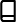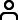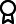Journal metrics
Acceptance rate14%
Submission to final decision40 days
Acceptance to publication54 days
CiteScore1.200
Journal Citation Indicator-
Impact Factor-

### Article of the Year 2020

The Role of Control Measures and the Environment in the Transmission Dynamics of Cholera

####Journal profile

Abstract and Applied Analysis publishes research with an emphasis on important developments in classical analysis, linear and nonlinear functional analysis, ordinary and partial differential equations, optimisation theory, and control theory.

####Editor spotlight

Chief Editor, Dr Wong, is an associate professor at Nanyang Technological University, Singapore. Her research interests include differential equations, difference equations, integral equations, and numerical mathematics.

####Special Issues

Do you think there is an emerging area of research that really needs to be highlighted? Or an existing research area that has been overlooked or would benefit from deeper investigation? Raise the profile of a research area by leading a Special Issue.

## Latest Articles

More articles
Research Article

### A Positive Answer on Nirenberg’s Problem on Expansive Mappings in Hilbert Spaces

Nirenberg proposed a problem as to whether or not a continuous and expansive operator (where is a Hilbert space) is surjective if . I shall give a positive answer for the problem provided that is unbounded. For contents related to this paper, the reader is referred to the remarks and the study of Asfaw (2021). The present paper gives a complete answer for the problem that has been open for about 47 years.

Research Article

### Common Fixed Point Theorem for Multivalued Mappings Using Ternary Relation in -Metric Space with an Application

This paper proves a common fixed point theorem for single-valued and multivalued mappings by using ternary relation in -metric spaces. Henceforth, results obtained will be verified with the help of illustrative examples. Also, we demonstrate the results with an application.

Research Article

### Strong Convergence Theorem for Finding a Common Solution of Convex Minimization and Fixed Point Problems in CAT(0) Spaces

In this paper, we introduce a proximal point algorithm for approximating a common solution of finite family of convex minimization problems and fixed point problems for -demicontractive mappings in complete CAT(0) spaces. We prove a strong convergence result and obtain other consequence results which generalize and extend some recent results in the literature. We further provide a numerical example to illustrate the convergence behaviour of the sequence generated by our algorithm.

Research Article

### Convergence of Mann and Ishikawa Iterative Processes for Some Contractions in Convex Generalized Metric Space

In this paper, we consider a JS metric space endowed with convexity structure, which will allow us to examine and study convergence of Mann iteration and Ishikawa iteration for Banach type and Chatterjea type contractions defined on JS metric space.

Research Article

### Preopenness Degree in -Fuzzy Bitopological Spaces

Based on the concept of pseudocomplement, we introduce a new representation of preopenness of -fuzzy sets in -fuzzy bitopological spaces. The concepts of pairwise -fuzzy precontinuous and pairwise -fuzzy preirresolute functions are extended and discussed based on the --preopen gradation. Further, we follow up with a study of pairwise -fuzzy precompactness in -fuzzy bitopological spaces of an -fuzzy set. We find that our paper offers more general results since -fuzzy bitopology is a generalization of -bitopology, -bitopology, and -fuzzy topology.

Research Article

### A New Class of Function with Finitely Many Fixed Points

The study introduced a generalized multiplier operator used as a tool to define and investigate a new class of function, and its subclass . Various properties of the class of functions were investigated. The results extend some known results in literature.Journal metrics
Acceptance rate14%
Submission to final decision40 days
Acceptance to publication54 days
CiteScore1.200
Journal Citation Indicator-
Impact Factor-Author guidelinesEditorial boardDatabases and indexingAuthor guidelinesEditorial boardDatabases and indexing

Article of the Year Award: Outstanding research contributions of 2020, as selected by our Chief Editors. Read the winning articles.